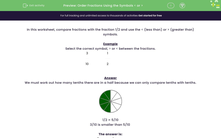# Order Fractions Using the Symbols < or >

In this worksheet, students compare fractions with the fraction 1/2 and use the < or > sign.Key stage:  KS 2

Curriculum topic:   Number: Fractions, Decimals and Percentages

Curriculum subtopic:   Order Fractions

Difficulty level:#### Worksheet Overview

In this worksheet, compare fractions with the fraction 1/2 and use the < (less than) or > (greater than) symbols.

Example

Select the correct symbol, > or < between the fractions.

 3 10
 1 2

We must work out how many tenths there are in a half because we can only compare tenths with tenths.1/2 = 5/10

3/10 is smaller than 5/10